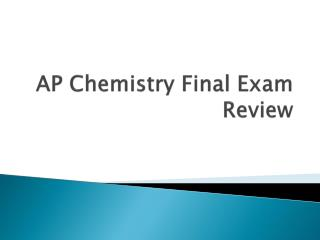DownloadDownload PresentationAP Chemistry Final Exam Review

# AP Chemistry Final Exam Review

Download Presentation## AP Chemistry Final Exam Review

- - - - - - - - - - - - - - - - - - - - - - - - - - - E N D - - - - - - - - - - - - - - - - - - - - - - - - - - -
##### Presentation Transcript

1. AP Chemistry Final Exam Review

2. Chapter 1 Matter and Measurements

3. Important Terms • Atom • Element • Law Of Definite Proportions

4. Mixture • Solution • Physical Properties • Chemical Properties

5. Intensive Property • Extensive Property • SI units

6. Precision • Accuracy • Significant figures • Dimensional Analysis

7. Equations • Density = m/v • K = oC + 273

8. Things You Should Be Able To Do • Solve simple problems using dimensional analysis. • Convert between Celsius and Kelvin

9. Chapter 2 Atoms, Molecules, and Ions

10. Important Terms • Nucleus • Proton, Neutron, Electron • Atomic Mass Unit

11. Isotope • Group • Period • Metal, nonmetal, metalloid

12. Molecule • Compound • Empirical Formula • Molecular Formula

13. Ion • Ionic Compound • Polyatomic Ion

14. Equations • None

15. Things You Should Be Able To Do • Calculate number of subatomic particles in an atom based on basic information about AMU’s and the type of element the atom is. • Work with empirical and molecular formulas. • Name ionic compounds. • Determine the formula of an ionic compound when given the name.

16. Example Problems • 20, 22, 47, 53, 69

17. Chapters 3 and 4 Stoichiometry

18. Important Words • Chemical Equation • Reactants • Products

19. Formula Weight • Mole • Molar Mass • Limiting Reactant

20. Theoretical Yield • Percent Yield • Aqueous solution • Sovlent

21. Solute • Electrolyte • nonelectrolyte • Strong and weak electrolyte

22. Precipitation Reaction • Molecular Equation • Complete Ionic Equation • Net Ionic Equation

23. Acid • Base • Neutralization Reaction • Oxidation • Oxidation state

24. Reduction • Concentration • Titration • Equivalence Point

25. Equations • Molarity = moles/L • M1V1 = M2V2

26. Things You Should Be Able To Do • Balance Equations • Calculate Molar Masses • Convert between moles and mass • Convert between Mass, moles, and number of particles. • Determine percent composition • Determine empirical and molecular formulas from percent composition data. • Calculate percent yeild

27. Example Problems • 1, 8, 9, 19, 21, 25, 41, 44, 63, 68, 74

28. Chapter 5 and 19 Thermodynamics and Thermochemistry

29. Important Terms • Thermodynamics • Thermochemistry • Kinetic Energy

30. Potential Energy • Joule • Calorie • System

31. Surroundings • Heat • First Law Of Thermodynamics • Endothermic

32. Exothermic • Enthalpy • Calorimetry • Heat Capacity

33. Molar Heat Capacity • Specific Heat • Hess’s Law • Enthalpy Of Formation

34. Standard Enthalpy of Reaction • Standard Enthalpy of Formation • Spontaneous • Isothermal

35. Second Law Of Thermodynamics • Third Law Of Thermodynamics • Gibbs Free Energy • Standard Free Energy of Formation

36. Equations • q = C xmx ΔT • ΔHorxn = sum(nΔHof products) – sum(ΔHof reactants) • Δso = sum(nΔS products) – sum(nΔS reactants) • ΔG = ΔH - TΔS • ΔGorxn = sum(nΔGof products) – sum(ΔGof reactants) • ΔG = ΔGo + RT lnQ • ΔGo =- RT lnk

37. Things You Should Be Able To Do • Understand the mathematical signs associated with thermodynamics. • Use Hess’s law to determine the heat energy transfer during a chemical reaction. • Calculate the heat transferred in a process using calorimetry. • Use standard enthalpies of formation to determine the standard enthalpy of a reaction. • Qualitatively describe entropy and entropy changes.

38. Calculate the standard entropy change for a process using standard molar entropies. • Calculate gibbs free energy from the enthalpy and entropy changes at a given temperature. • Predict the effect of temperature on spontaneity given ΔH and ΔS. • Calculate ΔG under nonstandard conditions. • Relate Δgo and equilibrium constants.

39. Example Problems • 29, 37, 46, 52,

40. Chapter 6 Electronic Structure Of Matter

41. Important Terms • Electron Configuration • Ground State • Excited State • Pauli exclusion principle

42. Hunds Rule • Valance Electrons

43. Equations • None

44. Things You Should Be Able To Do • Be able to interpret electron energy level diagrams. • Be able to write electron configurations.

45. Example Problems • 23

46. Chapter 7 Periodic Properties Of The Elements

47. Important Terms • Effective Nuclear Charge • Atomic Radius • Isoelectronic

48. Ionization Energy • Electron Affinity • Metallic Character

49. Equations • None

50. What You Should Be Able To Do • Understand how effective nuclear charge affects first ionization energy. • Use the periodic table to predict atomic radii, ionic radii, ionization energy, and electron affinity. • Understand how the ionization energy changes as we remove successive electrons.